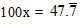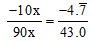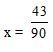# GEOMETRICAL REPRESENTATION OF REAL NUMBERS

## Number system of Class 9

To represent any real number of number line we follows the following steps :

Length BD is equal to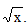.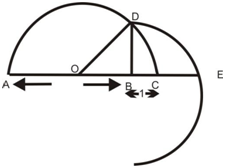### REPRESENTATION OF NUMBERS ON THE NUMBER LINE BY MEANS OF MAGNIFYING GLASS:

Method to find such numbers on the number line

1. Choose the two consecutive integral numbers in which the given number lies.

2. Choose the two consecutive decimal points in which the given decimal part lies by dividing the two given decimal parts into required equal parts.

3. Visualize the required number through magnifying glass.

For example:

Visualize the representation of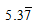on the number line upto 5 decimal places.

 Since 5 < 5.37777 < 6 5.3 < 5.37777 < 5.4 5.37 < 5.37777 < 5.38 5.377 < 5.37777 < 5.378 Proceeding by successive magnification and successively decreasing the lengths of the portions of the number line in whichis located. First, we observe thatis located between 5 and 6. In the second step, we locatebetween 5.3 and 5.4. To get a more accurate visualization, we divide this portion of the number line into 10 equal parts and use a magnifying glass to visualize thatlies between 5.37 and 5.38. Now to visualizestill more accurately, we divide the portion between 5.377 and 5.378 into 10 equal parts, and visualize the representation ofas shown in figure.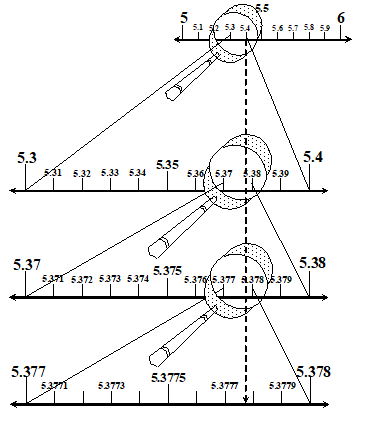### CONVERSION OF REPEATING DECIMAL INTO RATIONAL NUMBER:

For example:

Express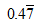in the form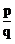.

Let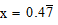Multiplying both sides by 10, we get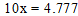…(i)

Multiplying both sides again by 10, we get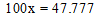…(ii)

Subtracting (i) from (ii), we get